Maths-
General
Easy

Question

# The sides of triangle are 11 cm, 15 cm and 16 cm. What is the measure of altitude to the largest side?Hint:

## The correct answer is: 2.64

### It is given that a = 11 cm , b = 15 cm and c = 16 cm.Using Heron’s formulaArea of triangle =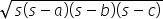where s =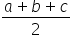s =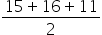= 21                                                              Area of triangle=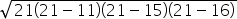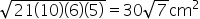Since we have to find altitude to the largest side, base of the triangle = 16 cmAlso, area of triangle =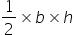⇒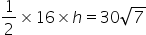⇒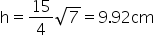(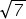= 2.64)#### With Turito Foundation.#### Get an Expert Advice From Turito.